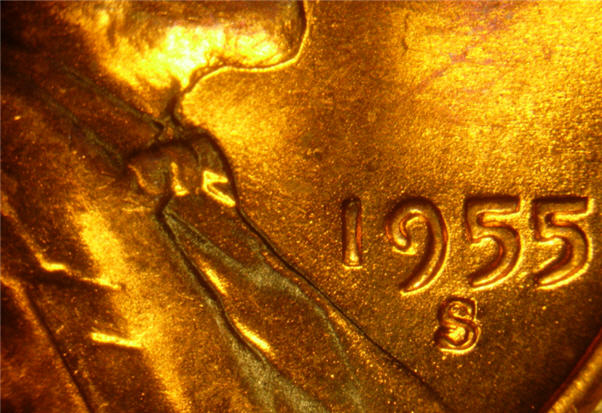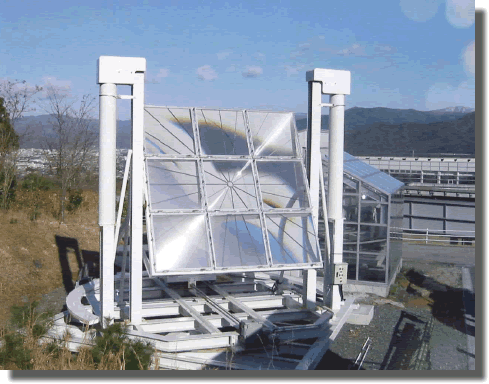# Poor man's arbitrageYou've found a stockpile of 1955 United States pennies, which are now worth approximately twice as much as raw metal compared to their value as currency. A Fresnel lens can be used to concentrate incoming light onto a focal point.For how many seconds would you need to focus the Fresnel lens on a penny in order to melt the entire coin?

Details and assumptions:

• A penny weighs $2.5 \text{ g}$ and is made of $100$% $\text{Cu}.$
• The penny starts out at $25^\circ\text{C}$ and melts at $1085^\circ\text{C}.$
• $\text{Cu}$'s heat of melting is $176 \text{ kJ}/\text{kg}$ and its specific heat is $0.386\text{ kJ}/\text{kg}\cdot\text{K}.$
• The area of the lens is $0.2 \text{ m}^2,$ the power of the sun is $1370\text{ W/m}^2,$ and $100$% of the energy goes into heating the coin.
×